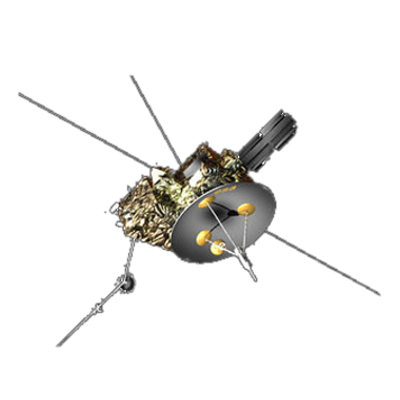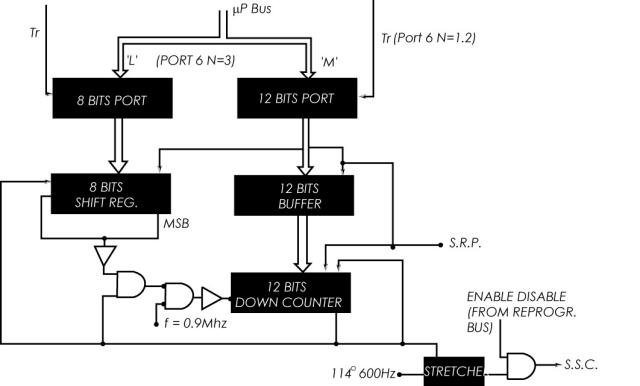ULYSSES

Ulysses HISCALE Data Analysis Handbook

4.7 Spin Reference Pulse

4.7.5 Onboard Generation of the Spin Segment Clock

The Spin Segment Clock (SSC) consists of 16384 pulses per spin period. The accuracy required is of plus or minus 6 pulses. The implementation is done by generating an SSC pulse after counting down M pulses at a frequency F.

The value of M is derived from the computed value of the SRP period and has a value equal to

M = Period*F/16384

The maximum frequency that could be used (technological considerations) is 917504 Hz. The actual time between two SRP, Q, is stored as a value on datation units (1/2048 sec.), and therefore the value of M is computed as

M = 7*Q/256

The value of M must be an integer number since it is used for loading a digital counter. This is the case for the nominal 5 rpm where the value of M is 672. In other cases where the value of M is not a pure integer, the error will be, in general, bigger than the requirements. For instance, if the period is 12.0178 sec. the value of M still will be 672 but the number of SSC pulses within two SRP will be

N = F* 12.0178/672 = 16408

The maximum error with this approach will be 24 SSC pulses. By rounding off the result of the division, the error will be plus or minus 12, still outside the requirements. Since F is fixed, the solution adopted consisted of varying the duration of the individual SSC pulses in such a way that the error is lower than 6 pulses. The implementation consists of loading an 8 bit shift register containing the remainder of the division. The register shifts with every SSC pulse and disables the first count if the bit is set and in practice stretches the width of the next SSC pulse by one count.

For example, if the value of M is 672 + 3/8, the shift register is loaded with 3, and of each 8 consecutive SSC's 5 will last 672 counts and 3 will last 673 counts, the mean being 672.375 and the number of SSC pulses during this spin period exactly 16384.

The diagram for the generation of the SSC is given in Figure 4.53. Table 4.17 gives the values of M, the SSC duration, and the maximum error for several spin rates.

Figure 4.53 Implementation of the SSCTable 4.17 Error on SSC

 rpm M Pulse Duration (μsec) Pulse Period SSC Error 3 1120 1220.7 ±0.9 1221.8 5 672 732.4 ±1.5 733.5 20 3 183.1 ±6.1 184.2